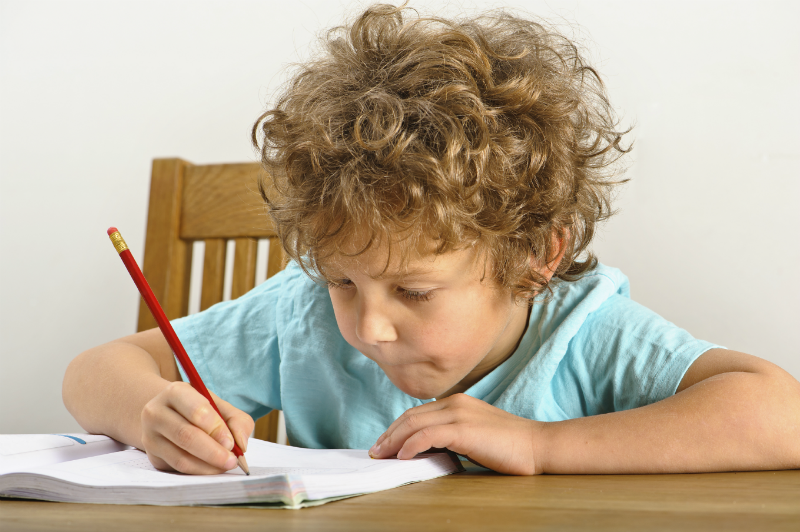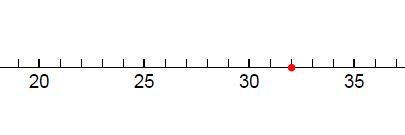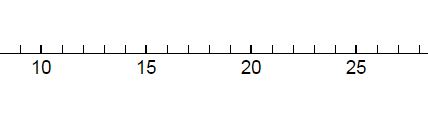# 10 essential maths skills for 7 year olds

This is a maths skills checklist for children reaching the end of Year 2 (or P3 in N Ireland) and is written to help parents ensure that the early signs of falling behind don't go unnoticed, so maths confidence can be quickly restored.

We've also developed a series of fun maths quizzes which are free and fun for kids to use and give parents private feedback on their child's strengths and identifies any areas which could benefit from a little extra attention. So why not take a Kickstart Quiz? (once you've read the article, of course!)

1) Number: Know one more or less than and ten more or less than any number from 1 to 100

"Write down the number 67 now write the number that is 10 more than this."

"What about the number that is one less, ten less."

2) Number: Count forward and backward in twos, fives and tens

"Starting at 12 count forward in twos"    "Starting at 60 count backwards in tens"

3) Number: Mark and read a number on a number line:

"What number is this?""Mark where the number 23 goes on this number line:"4) Number: Use place value to 100 ( tens and ones )

"What does the four stand for in 48?"

5) Number: Compare and order numbers to 100

"Put these numbers in order  27  56  23 78 14  smallest first"

6) Addition & Subtraction: Add and subtract to 20 ( including bridging 10 )

"7 + 8 = "           "17 + 9 = "

7) Addition & Subtraction: Missing numbers to 10

" 5 +  __ = 9   what's the missing number?"

8) Multiplication: Find double and half of a number

"Double 7 is "     "Half of 22 is"

9) Multiplication: Use multiplication as “lots of” and that the answer is the same when you change the order

"Two lots of three is?"            "Three lots of two is?"10) Multiplication: Know 2, 5 and 10 times tables

"3 x10 = "   "4 x 5 = "    "6 x 2 = "

A good indication your child has really mastered a skill is if they can answer correctly and quickly. Being hesitant is an indication that mastery isn't quite there.

The good news for parent and learners is that addressing problems in maths is easy when children are young, and in many cases all that's needed is some regular targeted practice.

I’m Ged, Co-founder of Komodo, ex-maths teacher and dad. If you have any questions please get in touch.

About Komodo – Komodo is a fun and effective way to boost primary maths skills. Designed for 5 to 11 year olds to use in the home, Komodo uses a little and often approach to learning maths (15 minutes, three to five times per week) that fits into the busy routine. Komodo users develop fluency and confidence in maths – without keeping them at the screen for long.

And now we've got Komodo English too - check it out here.

## Related Posts

### Mindset - the path to mastery

People who have a growth mindset believe that they always have the potential to learn and improve. They are more motivated to persevere with difficult tasks, to take risks and to learn from failure.

### Top tips to de-stress homework time

Homework can be a trigger for some of the worst fights and arguments that we have with our children. Here are some solutions that can help reduce tensions and get homework done without arguments.